# Bio Chem - Exam 1 Flashcards

Set Details Share
created 2 years ago by Lauren_Grabeau
152 views
updated 2 years ago by Lauren_Grabeau
Page to share:
Embed this setcancel
COPY
code changes based on your size selection
Size:
X

1

A polar molecule is one that has

a. a more positive side and a more negative side

b. an even distribution of charge

c. one large atom and one small atom

d. a positive or negative charge

e. only polar atoms

a. a more positive side and a more negative side

2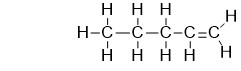Which is an adequate description of this hydrocarbon?

a. it is a polyunsaturated hydrocarbon

b. it is a multi unsaturated hydrocarbon

c. it is a saturated hydrocarbon

d. it is an unsaturated hydrocarbon

d. it is an unsaturated hydrocarbon

3

Compounds that are used as fuels

a. contain weak bonds

b. contain C--C bonds

c. contain C--H bonds

d. are high in potential energy

e. all of these are used a fuels

e. all of these are used as fuels

4An overview of metabolism is shown in the following figure. What does this figure tell us about the energy of catabolism and anabolism?

a. catabolism is exothermic, and anabolism is endothermic

b. overall, we every more energy than we consume

c. anabolic reactions occur more quickly than catabolic reactions

d. overall, the amount of energy that goes into anabolic processes is the same as is released in catabolic processes

e. the conservation of energy does not apply to biological systems

a. catabolism is exothermic, and anabolism is endothermic

5

What os the BEST name for the compound SF6, a potent greenhouse gas?

a. sulfur pentafluoride

b. sulfur fluoride

c. monosulfur fluoride

d. sulfur hexafluoride

e. sulfur fluorine

d. sulfur hexafluoride

6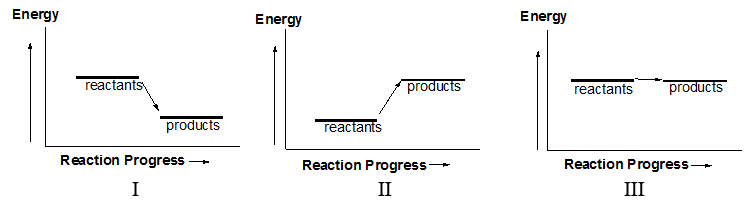Which of the following diagrams illustrates an exothermic reaction?

a. II only

b. I only

c. II and III

d. I and II

e. I and III

b. I only

7

_______________ are the subatomic particles that have the smallest mass.

a. Protons

b. Electrons

c. Neutrons

d. Protons and neutrons

e. Protons, neutrons, and electrons

b. Electrons

8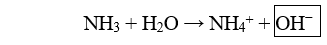The boxed species in the following reaction is

a. a hydronium ion

b. the conjugate acid

c. a proton

d. the acid

e. a hydroxide ion

e. a hydroxide ion

9

The identity of an element is determines by its number of ________________.

a. protons, neutrons, and electrons

b. protons and neutrons

c. neutrons

d. electrons

e. protons

e. protons

10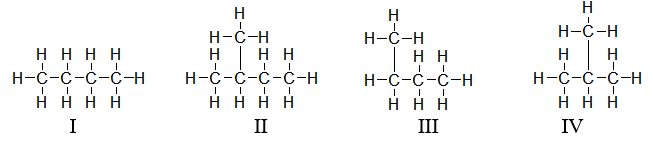Which structures are straight-chain hydrocarbons?

a. I and III

b. II and IV

c. I only

d. II, III, and IV

e. All of these molecules have a straight chain

a. I and III

11

Which of the following definition BEST describes structural isomers?

a. They are molecules that differ by carbon-carbon bond rotation

b. They are molecules that have the same molecular formula but different atom connectivity

c. They are molecules with the same molecular formula and the atom connectivity

d. They are molecules with different atom connectivity

e. They are molecules with the same physical properties

b. They are molecules that have the same molecular formula but different atom connectivity

12

Which of the following statements BEST describes what happens when an acid reacts with water?

I. The acid donates a proton.

II. Water donates a proton.

III. Water acts as a base.

IV. Hydronium is formed.

V. Hydroxide is formed.

a. I, III, and V

b. II and V

c. I, III, and IV

d. I only

e. II only

c. I, III, and IV

13

The nucleus is composed of _______________.

a. electrons

b. neutrons

c. protons, neutrons, and electrons

d. protons

e. protons and neutrons

e. protons and neutrons

14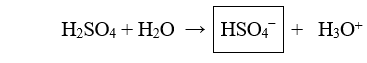The boxed species in the following reaction is

a. the conjugate base

b. a hydronium

c. a proton

d. a hydroxide

e. the acid

a. the conjugate base

15

A hydrogen atom without an electron is referred to as

a. hydronium

b. a proton

c. a conjugate base

d. an acid

e. hydroxide

b. a proton

16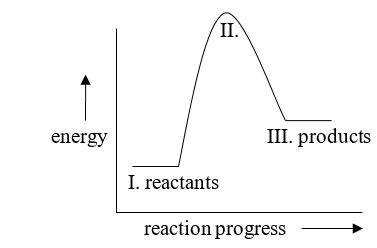Which part of the following energy diagram is changed when a catalyst is added, and how is it changed?

a. II is higher

b. II is lower

c. I is higher

d. III is higher

e. I is lower

b. II is lower

17

A conjugate acid-base pair is

a. the products of an acid-base reaction

b. two species that differ only by a proton

c. the reactants in an acid-base reaction

d. two species that differ only by a hydroxyl group

e. a single molecule that can act as both an acid and a base

b. two species that differ only by a proton

18

Which element is the MOST electronegative?

a. carbon

b. sulfur

c. nitrogen

d. oxygen

d. oxygen

19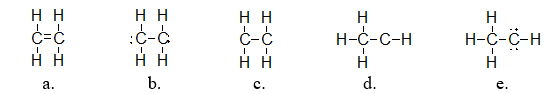Ethylene is used a a starting material in making plastics. It has a molecular formula of C2H4-. Which of the following structures is the correct Lewis structure for ethylene?

a. structure a

b. stricture e

c. structure b

d. structure d

e. structure c

a. structure a

20

Which of the following ions os a polyatomic ion?

a. O2-

b. NH4 +

c. O2

d. Mg2+

b. NH4 +

21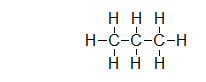Propane is a fuel commonly used in barbecues and to heat homes. It has the structure shown below. Does propane have a permeant dipole?

a. Yes. One side of the molecule is always partially positive, and the other side is partially negative

b. Yes. The carbon is partially positive, and the hydrogen is partially negative

c. Yes. The carbon is partially negative, and the hydrogen is partially positive

d. No. Propanse has a temporary dipole

e. No. Propane never displays any dipole at all

d. No. Propane has a temporary dipole

22

The only interactions between two or more molecules of a non polar material such as methane (CH4) are ________________ because these molecules do not have permanent dipoles.

a. covalent bonding

b. dispersion forces

c. dipole-dipole forces

d. ionic bonding

e. hydrogen bonding forces

b. dispersion forces

23

Which of the following statements about acids is FALSE?

a. an acid increases the concentration of protons in solution

b. an acid is a proton donor

c. an acid increases the concentration of hydronium in solution

d. an acid increases the concentration of hydroxide in solution

d. an acid increases the concentration of hydroxide in solution

24

When MgCl2 dissolves in water, it produces Mg2+ and Cl- ions. Which statement BEST describes the interaction between Cl- and water?

a. Chloride anions are attracted to the partial positive charge of hydrogen

b. Chloride anions are attracted to the partial negative charge of hydrogen

c. Chloride anions are attracted to the partial positive charge of oxygen

d. Chloride anions are not attracted to any part of water

e. Chloride anions are attracted to the partial negative charge of oxygen

a. Chloride anions are attracted to the partial positive charge of hydrogen

25

The following is the reaction of propanoic acid in water. Which choice is a conjugate acid-base pair in this reaction?

C3H5O2H (aq) + H2O (l) ⟷⟷ C3H5O2 (aq) + H3O+(aq)

propanoic acid propanoate

a. propanoate and water

b. propanoic acid and hydronium

c. propanoate and hydronium

d. propanoic acid and water

e. propanoic acid and propanoate

e. propanoic acid and propanoate

26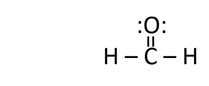Does formaldehyde have a permanent dipole?

a. Yes. The carbon is partially positive and the oxygen is partially negative

b. No. Formaldehyde only has a temporary dipole

c. Yes. The carbon is partially negative and the oxygen is partially positive

d. Yes. The carbon is partially negative and the hydrogen is partially positive

e. Yes. The carbon is partially positive and the hydrogen is partially negative

a. Yes. The carbon is partially positive and the oxygen is partially negative

27

Carbon tetrafluoride is a refrigerant and potent greenhouse gas. Which of the following is the molecular formula for this component.

a. CF4

b. C(IV)F

c. C4F4

d. CF

e. C4F

a. CF4

28

How many valence electrons are there in an oxygen atom?

a. 6

b. 2

c. 4

d. 8

a. 6

29

Match the atomic symbol and ion symbol with the correct terms.

Mg2+ Cl- Cδ+ F δ-

a. cation anion cation anion

b. cation anion partially+ C partially- F

c. anion cation anion cation

d. cation anion partially- C partially+ F

e. anion cation partially- C partially+ F

a. choice a

b. choice e

c. choice c

d. choice b

e. choice d

d. choice b

30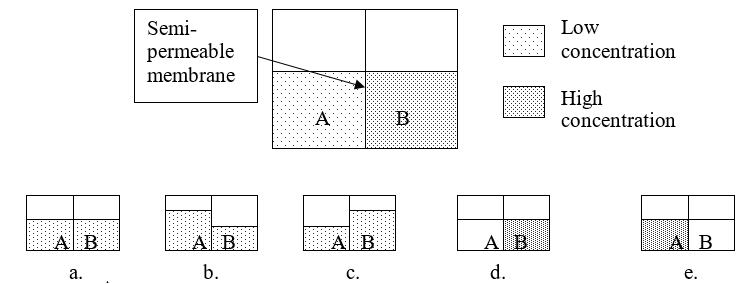Consider solution A and B separated by a semipermeable membrane. Which diagram BEST illustrates what the volumes should look like after osmosis occurs?

a. figure c

b. figure a

c. figure d

d. figure e

e. figure b

a. figure c

31

Which of the following changes increases the rate of a reaction?

b. decreasing concentration

c. removing product as it is formed

d. decreasing temperature

e. all of the above increase the rate of a reaction

32

A cation has _____________ electrons and is _____________ charged.

a. gained; positively

b. lost; positively

c. the same number of protons; not

d. gained; negatively

e. lost; negatively

b. lost; positively

33

Metabolism is

a. the tendency to gain or lose weight

b. the process by which fat is stored or burned

c. the citric acid cycle

d. the breakdown of food

e. all catabolic and anabolic pathways in the body

e. all catabolic and anabolic pathways in the body

34

Electronegativity is a measure if an atom's ability to

a. ionize

b. accept an electron from a metal

c. draw electrons to itself in a covalent bond

d. donate an electron to a nonmetal

e. become and anion

c. draw electrons to itself in a covalent bond

35

What buffer system is the primary buffering system in the blood?

a. DNA and RNA

b. H2CO3/HCO3 -

c. proteins

d. H2PO4 -/HPO4 2-

b. H2CO3/HCO3 -

36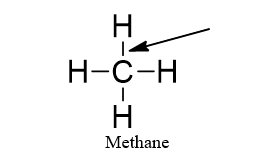The arrow is pointing to a(n)

a. triple bond

b. single bond

c. valence shell

d. double bond

e. electron 3

b. single bond

37

The reaction between acetic acid and water is given below, followed by a list of changes that could be made to the reaction solution. Which change will result in the equilibrium shifting to the left?

CH3COOH + H2O ⟷⟷ CH3COO + H3O+

Changes that could be made to the solution
II. Removing H2O
III. Removing H3O+

a. only IV will result in the equilibrium shifting to the left

b. only I will result in the equilibrium shifting to the left

c. I and III will result in the equilibrium shifting to the left

d. II and IV will result in the equilibrium shifting to the left

e. all of these changes will result in the equilibrium shifting to the left

d. II and IV will result in the equilibrium shifting to the left

38

A hydrogen atom without an electron is referred to as

a. a proton

b. an acid

c. hydronium

d. hydroxide

a. a proton

39

How do strong and weak acids differ?

a. strong acids ionize completely in aqueous solution and weak acids don't

b. only strong acids produce hydronium in aqueous solutions

c. strong acids increase the concentration of OH- in solution

d. strong acids donate protons and weak acids do not

a. strong acids ionize completely in aqueous solution and weak acids don't

40What is the strongest type of molecular interaction attracting molecules of propane together?

a. dipole-dipole forces

b. hydrogen bonding forces

c. dispersion forces

d. covalent bonding

e. ionic bonding

c. dispersion forces

41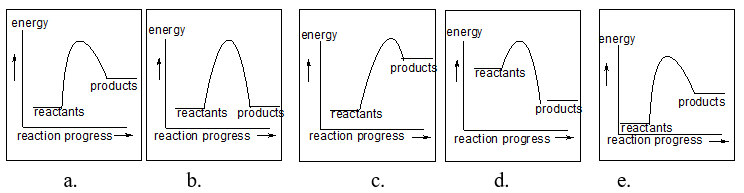Each of the following figures represents a reaction. Which reaction(s) has/have the slowest rate?

a. a, b, c, d, and e

b. a and b

c. b and e

d. all but d

e. b only

d. all but d

42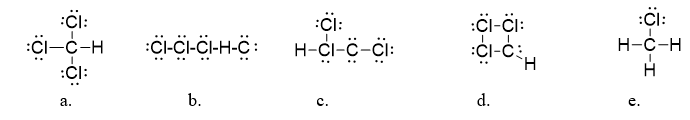Chloroform (CHCl3) is an anesthetic and is also used in synthesis of ozone-damaging refrigerants called CFCs. What is the correct Lewis dot structure for chloroform?

a. structure c

b. structure a

c. structure e

d. structure b

e. structure d

b. structure a

43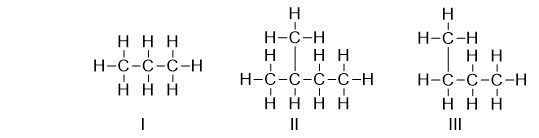Which of the following structures is a branched-chain hydrocarbon?

a. II only

b. II and III

c. I and II

d. III only

e. I only

a. II only

44

Which of the following interactions is the strongest?

a. dipole-dipole forces

b. dispersion forces

c. covenant bond

d. hydrogen bonding forces

e. all of the above interactions have the same strength

c. covalent bond

45

What is the chemical formula of the compound formed by sodium and oxygen?

a. Na2O3

b. NaO2

c. Na3O2

d. Na2O

e. NaO

d. Na2O

46

Electrons shared between two atoms is a(n) _________________.

a. covalent bond

b. cation

c. ionic bond

d. salt

e. anion

a. covalent bond

47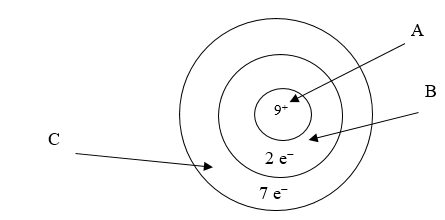Below is an illustration of an atom of fluorine. Which arrow points to the part of the atom involved in bonding?

a. B

b. A

c. both B and C point to the correct part of the atom

d. none of the above is involved in binding

e. C

e. C

48

What is the ration of ions in Li2O?

a. 2 Li : 1 O

b. 4 O : 5 Li

c. 4 Li : 5 O

d. 1 Li : 1 O

e. 2 O : 1 Li

a. 2 Li : 1 O

49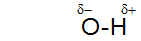What is meant by the following symbols?

a. This means the the oxygen has donated an electron to the hydrogen

b. These are not symbols used in chemistry

c. This means that the oxygen is pulling electrons towards itself and is partially negative

d. This means that the hydrogen has donated an electron to the oxygen

e. This means that the hydrogen is pulling electrons toward itself and is partially positive

c. This means that the oxygen is pulling electrons towards itself and is partially negative

50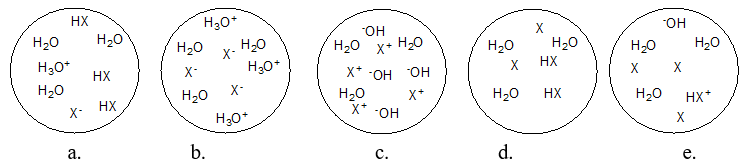Each circle is a sample of an aqueous acidic or basic solution. Which solution contains a strong acid?

a. figure c

b. figure b

c. figure d

d. figure a

e. figure e

b. figure b